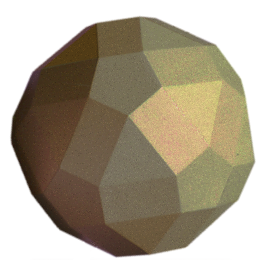# The Elongated Rhomb·icosi·dodecahedronThe rhombicosidodecahedron is the semi-regular polyhedron made up of 12 pentagons, 20 triangles, and 30 squares.  As you can see from the sketch on the right, the pentagons have a somewhat stronger visual impact than the other polygons.

Something that may not be immediately obvious is that the squares form a bridge between two regular polyhedrons, the dodecahedron and the icosahedron. Generalizing the squares to rectangles allows one to reduce the visual impact of the pentagons:In your mind, uniformly vary the shape of the rectangles.  As the length of the pentagonal sides of the rectangles approaches 0, the polyhedron converges to an icosahedron.  Similarly, if the length of the triangular sides is allowed to approach 0, the polyhedron converges to a dodecahedron.